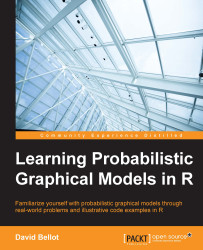•#### Learning Probabilistic Graphical Models in R#### Overview of this book

Probabilistic graphical models (PGM, also known as graphical models) are a marriage between probability theory and graph theory. Generally, PGMs use a graph-based representation. Two branches of graphical representations of distributions are commonly used, namely Bayesian networks and Markov networks. R has many packages to implement graphical models. We’ll start by showing you how to transform a classical statistical model into a modern PGM and then look at how to do exact inference in graphical models. Proceeding, we’ll introduce you to many modern R packages that will help you to perform inference on the models. We will then run a Bayesian linear regression and you’ll see the advantage of going probabilistic when you want to do prediction. Next, you’ll master using R packages and implementing its techniques. Finally, you’ll be presented with machine learning applications that have a direct impact in many fields. Here, we’ll cover clustering and the discovery of hidden information in big data, as well as two important methods, PCA and ICA, to reduce the size of big problems.
Learning Probabilistic Graphical Models in RCreditswww.PacktPub.comPrefaceFree Chapter
Probabilistic ReasoningExact InferenceLearning ParametersBayesian Modeling – Basic ModelsApproximate InferenceBayesian Modeling – Linear ModelsProbabilistic Mixture ModelsAppendixIndex## Mixture models

The mixture model is a model of a larger distribution family called latent variable models, in which some of the variables are not observed at all. The reason is usually to simplify the model by grouping all the variables into subgroups with a different meaning. Another reason is also to introduce a hidden process into the model, the real reason for the data generation process. In other words, we assume that we have a set of models and something hidden will select one of these models, and then generate a data point from the selected model.

When the data naturally exhibits clusters, it seems reasonable to say that each cluster is a small model.

The whole problem is then to find to what extent a submodel will participate in the data generation process and what the parameters for each sub model are. This is usually solved using the EM algorithm.

There are many ways to combine small models in order to make a bigger or more generic model. The approach generally used in mixture modeling...